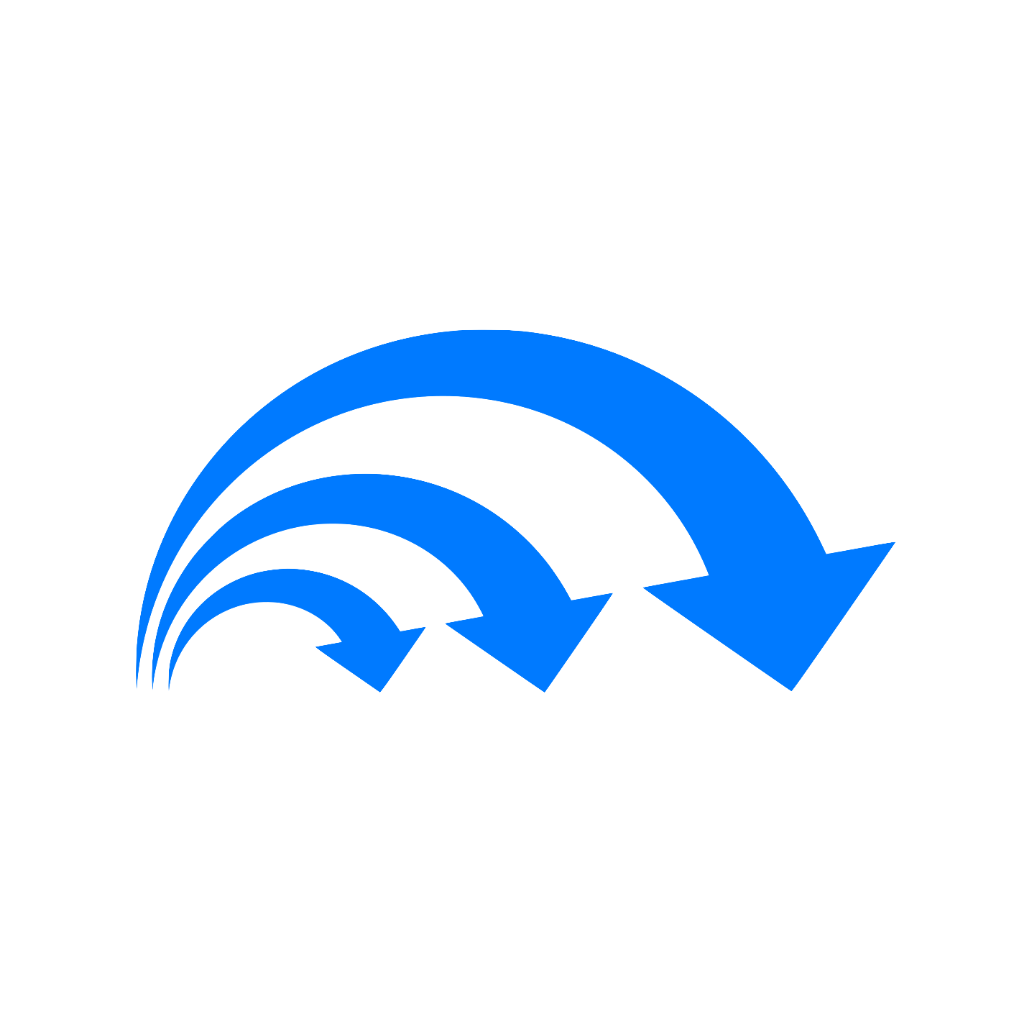# CSCI 163A - UNIT 1

Martin Rios-Cardenas (lvl 5)
Master Theorem

### Preview this deck

if $$a > b^d$$

Front### 0.0

0 reviews

 5 0 4 0 3 0 2 0 1 0

Active users

1

All-time users

2

Favorites

0

Last updated

3 years ago

Date created

Jan 27, 2021

## Cards(4)

Master Theorem

(4 cards)

if $$a > b^d$$

Front

$$T(n) \in \Theta(n^{\log_ab)$$

Back

if $$a < b^d$$

Front

$$T(n) \in \Theta(n^d)$$

Back

if $$a = b^d$$

Front

$$T(n) \in \Theta(n^d \log n)$$

Back

What is the Master Theorem look like?

Front

\begin{aligned} T(1) &= c \\ T(n) &= aT(n/b) + f(n) \end{aligned}

In order to find d, we need to find the highest power of $$f(n)$$

Back